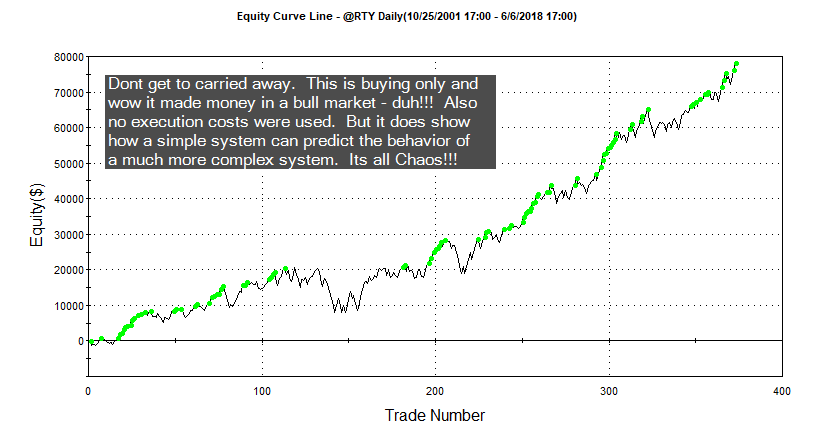# Update To Original Pattern Smasher

## What will you learn : string manipulation, for-loops, optimization

Before proceeding I would suggest reading my original post on this subject.    If you believe the relationship of the last few bars of data can help determine future market direction, then this post will be in you wheel house.  Another added benefit is that you will also learn some cool EasyLanguage.

### Original post was limited to four day patterns!

This version is limitless (well not really, but pretty close).  Let’s stick with the original string pattern nomenclature (+ + – – : two up closes followed by two down closes.)  Let’s also stick with our binary pattern representation:

 Pattern # 2^3 2^2 2^1 1 3 0 0 1 1 4 0 1 0 0 5 0 1 0 1 6 0 1 1 1

Remember a 0 represents a down close and a 1 represents an up close.  We will deviate from the original post by doing away with the array and stick with only strings (which are really just arrays of characters.)  This way we won’t have to worry about array manipulation.

#### How to create a dynamic length string pattern

This was the difficult part of the programming.  I wanted to be able to optimize 3, 4 and 5 day patterns and I wanted to control this with using just inputs.  I discovered that pattern three is different in a three day pattern than it is in a four day pattern: in a three day pattern it is 011 or – + + and in a four day pattern it is 0011 or – – + +.  Since I am counting 0’s as down closes, pattern #3 depends on the ultimate size of the pattern string.  No worries I will have eventually have another version where I utilize a different value for down closes and we can then have holes in our string patterns.  But I digress – so to differentiate the patterns based on the pattern length I included a maxPatternLen input.  So if maxPatternLen is three and we are trying to match pattern #3 then we will be looking for 011 and not 0011.  That was an easy fix.  But then I wanted to build a string pattern based on this input and the pattern number dynamically.  Here is some psuedo code on how I figured it out.

``````
{Psuedo code to translate pattern number into binary number}
patternNumber = 3
maxPatternLen = 3

numBits = 0    						// stick with binary representation
testValue = 0						// temporary test value
numBits = maxPatternLen-1  			// how many bits will it take to get to the
// center of - or numBits to represent max
// number of patterns or 2^numBits
currentBit =numBits					// start wit current bit as total numBits

value1 = patternOptTest				// value1 represents current pattern number
testString = ""  					// build test string from ground up

for icnt = numBits downto 0			//building string from left to right
begin       						// notice keyword downto
if power(2,currentBit) > value1 then  // must use power function in EL
begin							// if the very far left bit value >
testString = testString + "-"	  // patten number then plug in a "-"
end
else
begin							// else plug in a "+" and deccrement by
testString = testString + "+"	 // that bits value - if its the 3rd bit
value1 = value1 - power(2,currentBit)// then decrement by 8
end;
currentBit = currentBit - 1		// move onto the next bit to the right
end;``````
Pseudocode for Binary Representation of Pattern #

Now if you want to optimize then you must make sure your pattern number search space or range can be contained within maxPatternLen.  For example, if you want to test all the different combinations of a four day pattern, then your maxPatternLen would naturally be four and you would optimize the pattern number from 0 to 15.  Don’t use 1-16 as I use zero as the base.  A five day pattern would include the search space from 0 – 31.  The rest of the code was basically hacked from my original post.   Here is the rest of the code to do optimizations on different length pattern strings.  Notice how I use strings, for-loops and comparisons.

``````input: buyPattern("+++-"),sellPattern("---+"),patternOptimize(True),patternOptTest(7),maxPatternLen(3),patternOptBuySell(1),
stopLoss\$(2000),profitTarg\$(2000),holdDays(5);

sellPatternMatch = sellPattern;
numCharsInSellPattern = strLen(sellPatternMatch);

If patternOptimize then
begin
numBits = 0;
testValue = 0;
value = maxPatternLen;
numBits = maxPatternLen-1;
currentBit =numBits;
remainder = patternOptTest;
testString = "";
for icnt = numBits downto 0
begin
if power(2,currentBit) > remainder then {note this originally had value1 instead of remainder}
begin
testString = testString + "-";
end
else
begin
testString = testString + "+";
remainder = remainder - power(2,currentBit);
end;
currentBit = currentBit - 1;
end;
numCharsInSellPattern = maxPatternLen;
Begin
sellPatternMatch = "0";
end;
Begin
sellPatternMatch = testString;
end;
end;

sellPatternString = "";

For icnt = numCharsInBuyPattern-1 downto 0
Begin
end;
For icnt = numCharsInSellPattern-1 downto 0
Begin
If close[icnt] >= close[icnt+1] then sellPatternString = sellPatternString + "+";
If close[icnt] < close[icnt+1] then sellPatternString = sellPatternString + "-";
end;

okToSell = false;

If sellPattern <> "" then
If sellPatternString = sellPatternMatch then okToSell = true;
If sellPatternMatch = "" then
okToSell = true;

If okToSell then sellshort next bar at open;

If marketPosition = 1 and barsSinceEntry > holdDays then sell next bar at open;
If marketPosition = -1 and barsSinceEntry > holdDays then buytocover next bar at open;

setStopLoss(stopLoss\$);
setProfitTarget(profitTarg\$);